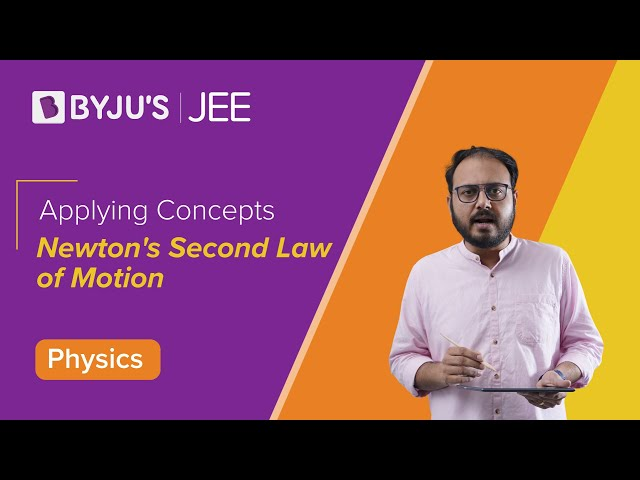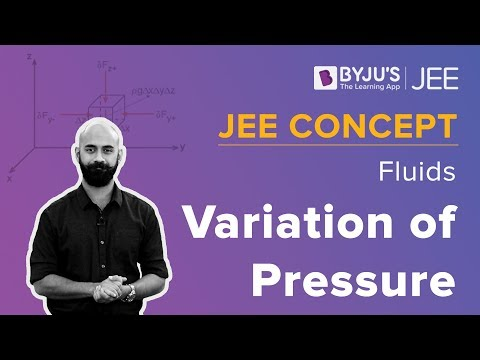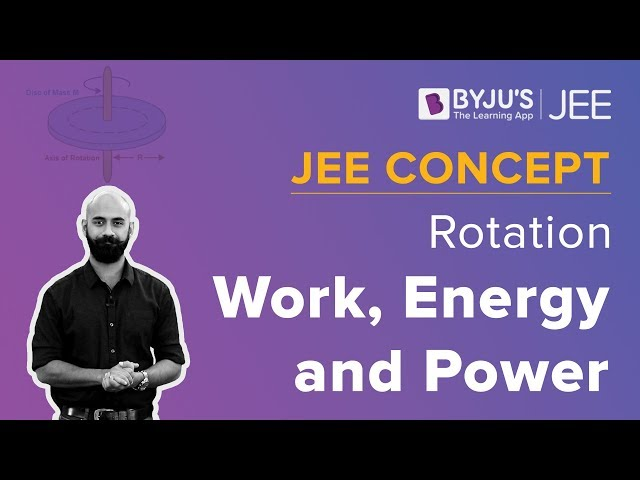Win up to 100% scholarship on Aakash BYJU'S JEE/NEET courses with ABNAT Win up to 100% scholarship on Aakash BYJU'S JEE/NEET courses with ABNAT

# JEE Main 2020 Chemistry (Shift 2-5th Sept) Paper With Solutions

To succeed in the JEE examination candidates need to prepare well and effectively. One way to study productively is by solving previous years’ question papers. IIT JEE aspirants can refer to this page for the JEE Main 2020 (Shift 2-5th Sept) Chemistry Question Paper with Solutions. These questions are solved by the experts at BYJU’S in the simplest possible method.
September 5 Shift 2 – Chemistry

1. The major product formed in the following reaction is: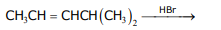1) CH3CH(Br)CH2CH(CH3)2
2) CH3CH2CH2C(Br)(CH3)2
3) CH3CH2CH(Br)CH(CH3)2
4) Br(CH2)3CH(CH3)2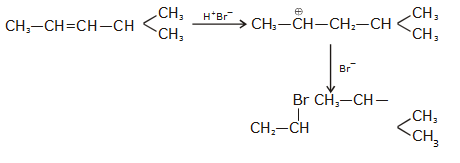2. Hydrogen peroxide, in the pure state, is:

1) Linear and blue in color
2) Linear and almost colorless
3) Non-planar and almost colorless
4) Planar and blue in color

H2O2 has open book structure it is non planar

3. Boron and silicon of very high purity can be obtained through:

1) Liquation
2) Electrolytic refining
3) Zone refining
4) Vapour phase refining

Fact

4. The following molecule acts as an: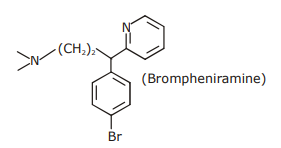1) Anti-histamine
2) Antiseptic
3) Anti-depressant
4) Anti-bacterial

Anti-histamine

5. Among the following compounds, geometrical isomerism is exhibited by: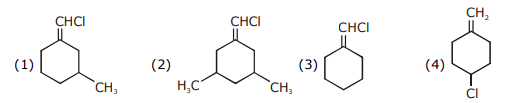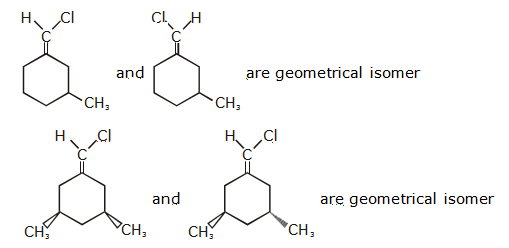6. Adsorption of a gas follows Freundlich adsorption isotherm. If x is the mass of the gas adsorbed on mass m of the adsorbent, the correct plot of x/m versus p is: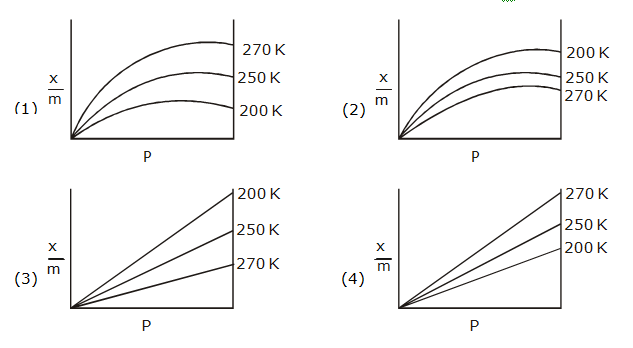As temp. increases extent of Adsorption decreases

Therefore correct option (2)

(x/m)= KP 1/n

(x/m) v/s P is non linear curve

7. The compound that has the largest H–M–H bond angle (M=N, O, S, C) is:

1) CH4
2) H2S
3) NH3
4) H2O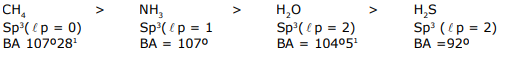8. The correct statement about probability density (except at infinite distance from nucleus) is :

1) It can be zero for 3p orbital
2) It can be zero for 1s orbital
3) It can never be zero for 2s orbital
4) It can negative for 2p orbital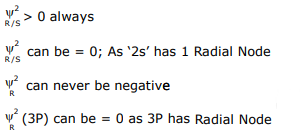9. The rate constant (k) of a reaction is measured at different temperatures (T), and the data are plotted in the given figure. The activation energy of the reaction in kJ mol–1 is: (R is gas constant)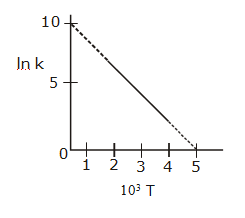1) R
2) 2/R
3) 1/R
4) 2R

$$\begin{array}{l}In(k)=In(A)-\frac{Ea}{R}\left [ \frac{1}{T} \right ]\end{array}$$

In(A)= 10

$$\begin{array}{l}Slope = \frac{-Ea}{R}\times 10^{-3}=-10/5\end{array}$$

Ea= 2000R J/mol

Ea= 2R KJ/mol

10. The variation of molar conductivity with concentration of an electrolyte (X) in aqueous solution is shown in the given figure.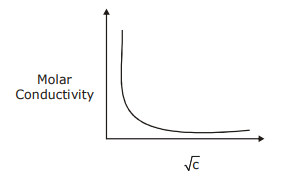The electrolyte X is:

1) HCl
2) CH+COOH
3) NaCl
4) KNO3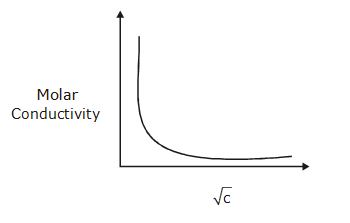Such type of variation is always for weak electrolyte. Hence Ans. (2) CH3COOH

11. The final major product of the following reaction is: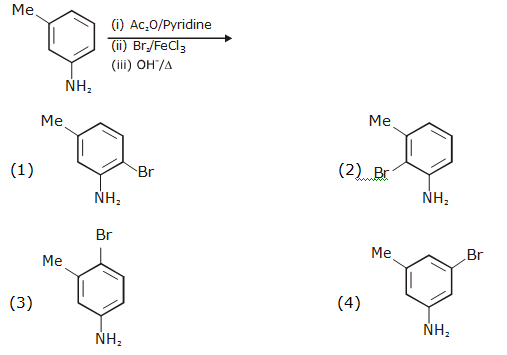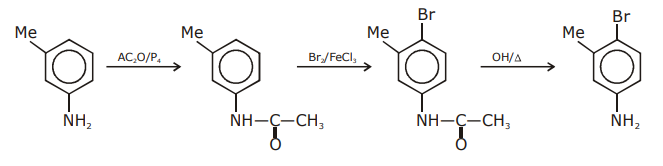12. The major product of the following reaction is :13. Lattice enthalpy and enthalpy of solution of NaCl are 788 kJ mol–1, and 4 kJ mol–1, respectively. The hydration enthalpy of NaCl is:

1) –780kJ mol–1
2) 784kJ mol–1
3) –784kJ mol–1
4) 780kJ mol–1

ΔHsol=L.E + ΔHhyd

4 = 788 + ΔHhyd

ΔHhyd = – 784 KJ/mol Ans

14. Reaction of ammonia with excess Cl2 gives:

1) NH4Cl and N2
2) NH4Cl and HCl
3) NCl3 and HCl
4) NCl3 and NH4Cl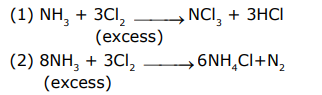15. Which one of the following polymers is not obtained by condensation polymerisation?

1) Bakelite
2) Nylon 6
3) Buna-N
4) Nylon 6, 6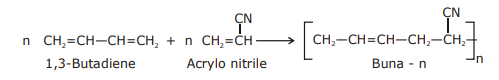16. Consider the comples ions, trans-[Co(en)2Cl2]+ (A) and cis-[Co(en)2Cl2]+ (B). The correct statement regarding them is:

1) Both (A) and (B) can be optically active.
2) (A) can be optically active, but (B) cannot be optically active.
3) Both (A) and (B) cannot be optically active.
4) (A) cannot be optically active, but (B) can be optically active.

Due to presence of Pos (A) cannot be optically active, but (B) can be optically active

17. An element crystallises in a face-centred cubic (fcc) unit cell with cell edge a. The distance between the centres of two nearest octahedral voids in the crystal lattice is:

1) a
2) a/2
3) √2a
4) a/√2

Nearest octahedral voids

One along edge center & other at Body centre

$$\begin{array}{l}Distance=\sqrt{\left [ \frac{a}{2} \right ]^{2}+\left [ \frac{a}{2} \right ]^{2}}=\sqrt{2}\frac{a}{2}=\frac{a}{\sqrt{2}}\end{array}$$

18. The correct order of the ionic radii of O2–, N 3–, F, Mg2+, Na+ and Al3+ is:

1) N3– < O2– < F < Na+ < Mg2+ < Al3+
2) N3– < F < O2– < Mg2+ < Na+ < Al3+
3) Al3+ < Na+ < Mg2+ < O2– < F < N3–
4) Al 3+ < Mg2+ < Na+ < F < O2– < N3–

All are Isoelectronic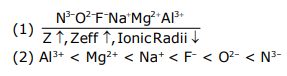19. The increasing order of boiling points of the following compounds is: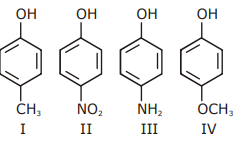1) I < III < IV < II
2) IV < I < II < III
3) I < IV < III < II
4) III < I < II < IV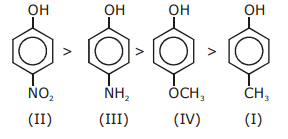20. The one that is NOT suitable for the removal of permanent hardness of water is:

1) Ion-exchange method
2) Calgon’s method
3) Treatment with sodium carbonate
4) Clark’s method

Clark’s method is used for Removal of Temporary hardness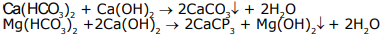21. For a reaction

$$\begin{array}{l}X+Y\rightleftharpoons 2Z\end{array}$$
, 1.0 mol of X, 1.5 mol of Y and 0.5 mol of Z were taken in a 1 L vessel and allowed to react. At equilibrium, the concentration of Z was 1.0 mol L–1. The equilibrium constant of reaction is ________ x/15. The value of x is _________.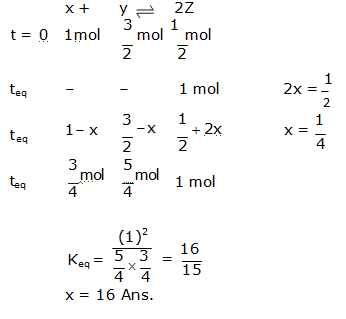22. The volume, in mL, of 0.02 M K2Cr2O7 solution required to react with 0.288 g of ferrous oxalate in acidic medium is ________. (Molar mass of Fe= 56 g mol–1)

K2Cr2O7+ FeC2O4 → Cr3+ + Fe3+ + CO2

$$\begin{array}{l}\frac{0.02\times vol\times 6}{1000}=3 \times \frac{0.288}{144}\times 100\end{array}$$

Vol. = 200/4 = 50 ml Ans.

23. Considering that ∆0 > P, the magnetic moment (in BM) of [Ru(H2O)6] 2+ would be ________.

[Ru(H2O)6)2+

Ru 2+ = 3d6(∆0 > P)

= t2g6 eg0

n = 0, u = 0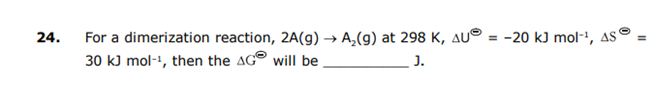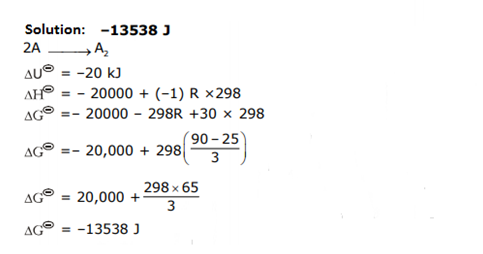25. The number of chiral carbons present in sucrose is ______.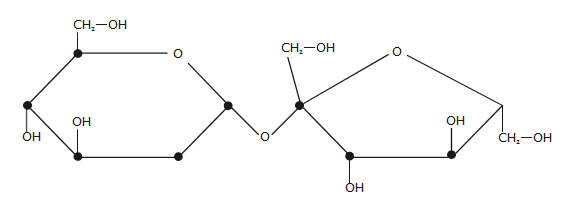## Video Lessons – September 5 Shift21 Chemistry